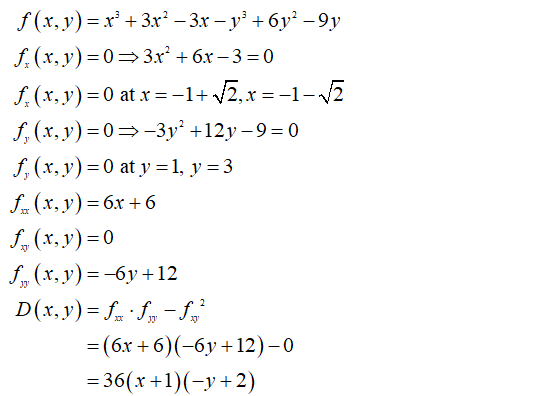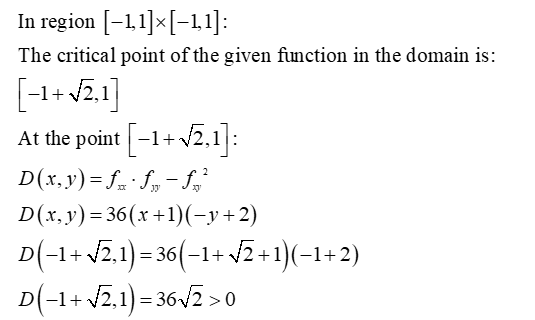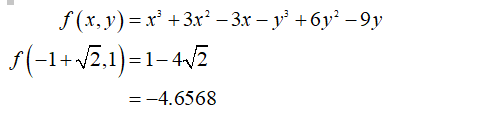# Find and classify all the critical points of f(x,y) = x3+3x2-3x-y3+6y2-9y.  Find the maximum and minimum of f on the region x[-1,1], y[-1,1]

Question
1 views

Find and classify all the critical points of f(x,y) = x3+3x2-3x-y3+6y2-9y.  Find the maximum and minimum of f on the region x[-1,1], y[-1,1]

check_circle

Step 1

Find partial derivatives of the function f (x, y) = x3 + 3x2 - 3x – y3 + 6y2 – 9y with respect to x  and y.Step 2

We have to find the maximum and minimum of function on the region x [-1,1], y [-1,1]:

There is only one point of the given domain which is a critical point:Step 3

This point is the minimum point of the function and the minimum value of the function is:...

### Want to see the full answer?

See Solution

#### Want to see this answer and more?

Solutions are written by subject experts who are available 24/7. Questions are typically answered within 1 hour.*

See Solution
*Response times may vary by subject and question.
Tagged in